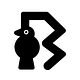# Elixir Intermediate — The Hard Code Behind Smooth Code

What you see is more simplified.

This article shows you the rigid lines of code behind smooth lines you write in elixir.

As we know, the most of the Elixir programming language is written in Elixir itself with the power of Macros and also we all know why we love to code in Elixir because of its simplicity. But, today we are going to walk back to find the light in the dark.

I just want you to observe the following line of code for a while

`if 1+2==3, do: :this, else: :that`

Here, I am not gonna ask you what this results to. But, I am asking you how this gonna transform eventually.

Let’s observe the transformations.

As we know the elixir allows the function call with out `()` . This is why the function calling is simple. But, I recommend to use `()` which is more understandable of what is going on.

If you observe above line, we have couple of `,` .

This is more likely `if` is taking three parameters `1+2==3` , `do: :this` and `else: :that` but, in real it is taking two.

The last two parameters `do: :this` and `else: :that` are together called a `KeyWord List` .

If you don’t know what a `keyWord List` is, It is likely a `list` where its list elements should be `tuples` of two elements where first element should be an `atom` .

In one sentence, `keyword list` is a list of tuples where tuple size should be two (2) and first element in a `tuple` is an `atom` . In real it is list of key-value pairs where key is an `atom` and value can be `any` .

Consider the following example

`iex> list = [{:a, 1}, {:b, 2}][a: 1, b: 2]iex> list == [a: 1, b: 2]true`

Here `[{:a, 1}, {:b, 2}] == [a: 1, b: 2]` . Enough with `keyword lists` .

Let’s jump back to our line of code

`if 1+2==3, do: :this, else: :that`

If the last parameter to pass is a `KeyWord List` , we no need to wrap elements inside the `[]` square brackets likely we do in general.

`hello("medium", user_name: "blackode", lang: "elixir")`

is same as

`hello("medium", [user_name: "blackode", lang: "elixir"])`

Here, both are considered as valid.

So, the line of code is transformed from

`if 1+2==3, [do: :this, else: :that]`

to

`if( 1+2==3, [do: :this, else: :that] )`

Now, observe the first parameter `1+2 == 3` which is `(1+2)==3` .

Here, before resolving `==` we have to add `(1+2)` .

The `(1+2) `is more likely a function with two parameters. It is an infix notation and `+` is called an infix operator.

It is a different way of doing certain functions where the function_name is in the middle. The left and right values are passed as first and second parameters respectively to the function.

So, the traditional syntax of representing `(1+2)` is `+(1,2)` .

Similarly we break down the another infix operator `==` as `==(+(1,2), 3)` just like we did for `+` nothing more fancy here.

Well, so far we have break down the `+(1,2)==3` to `==(+(1,2), 3)` .

Here, we cannot use the infix operators with out its module name where they are defined. All the operators are defined inside the module called `Kernel` . This is the default module in elixir.

So, again we break down `==(+(1,2), 3)` to `Kernel.==(Kernel.+(1,2), 3)`

Now, the line is transformed to as following

`if(Kernel.==(Kernel.+(1,2), 3), [do: :this, else: :that])`

We all know that `if` is a macro which is more likely represented `Kernel.if`

So, putting all together and breaking down the infix functions, the line again is transformed into

`Kernel.if( Kernel.==(Kernel.+(1,2), 3), [do: :this, else: :that ] )`

The best part is, the elixir compiler will take care of all these transformations.

We no need to write all the stuff like above.

Hope you got some idea about hotness behind the cool syntax. For deeper understanding, read more about the MACROS.

Happy Coding !!

Written by

Written by

## Blackode

#### coding, thoughts & ideas